Published © CC BY

# PID Battery Charger Controller

Test and comparison of two PWM methods, to control the battery charge of a "Night Light Control:" 1) Sine function; and 2) PID controller.

AdvancedFull instructions providedOver 8 days595

## Things used in this project

### Hardware components

 STM32F407G-DISC1
×1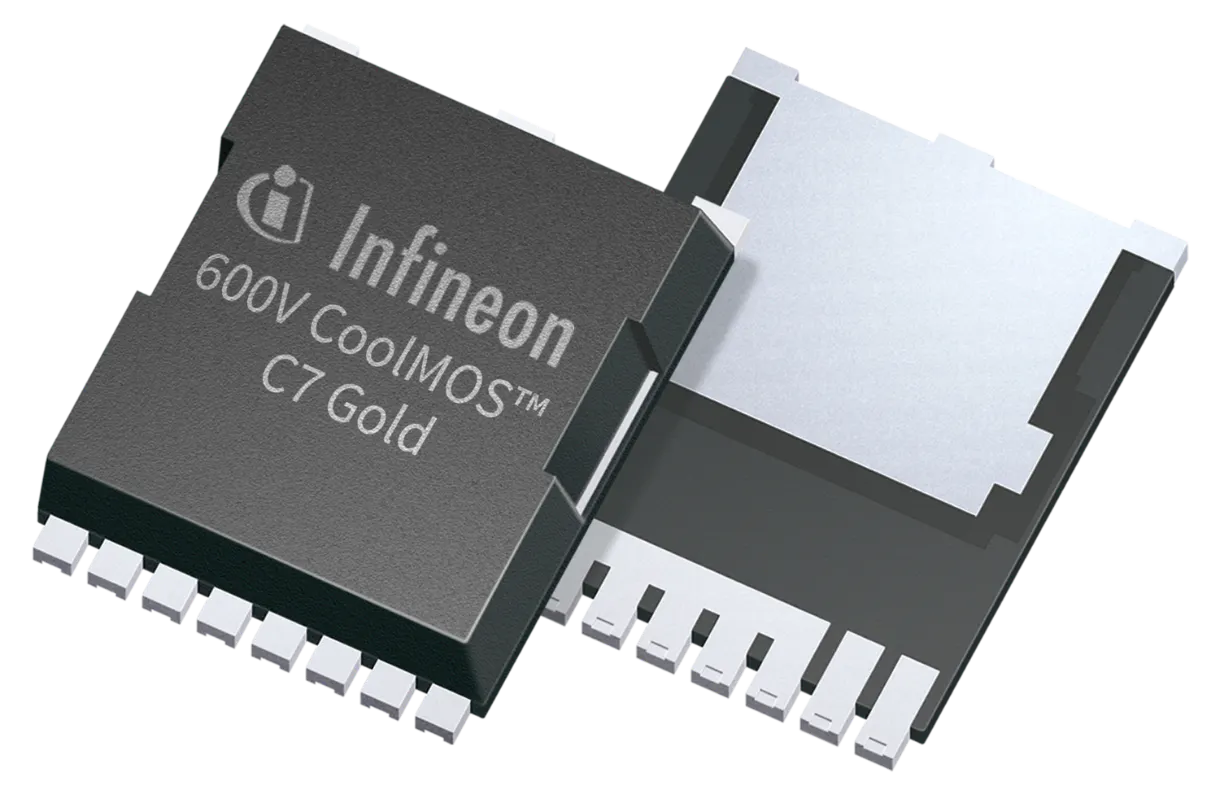Infineon CoolMOS C7 Gold SJ MOSFET
×1
 J525 P-Channel Mosfet (Toshiba)
×1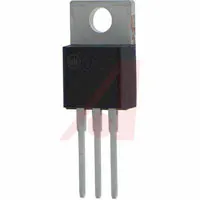Linear Regulator with Adjustable Output
×1
 BC547 - NPN Transistor
×3
 BD135 - NPN Transistor
×2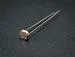Photo resistor
×1
 40106 (Trigger Schmitt)
×1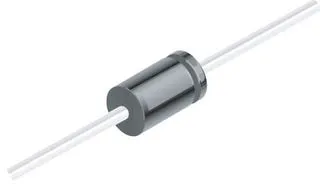1N4007 – High Voltage, High Current Rated Diode
×2
 Schottky diode
×1
 Rechargeable Battery 5-6 Volts
×1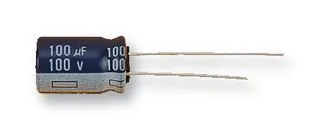Capacitor 100 µF
×1
 Capacitor .1 uF
×1
 Potentiometer 5k
×1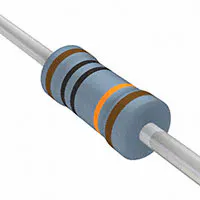Resistor 100k ohm
×1Resistor 10k ohm
×6
 Resistor 4k7
×2Resistor 1k ohm
×2
 Resistor 3k3
×1
 Resistor 240 ohm
×1
 Zener diode - 11v
×1
 Fuse (5A)
×2

### Software apps and online services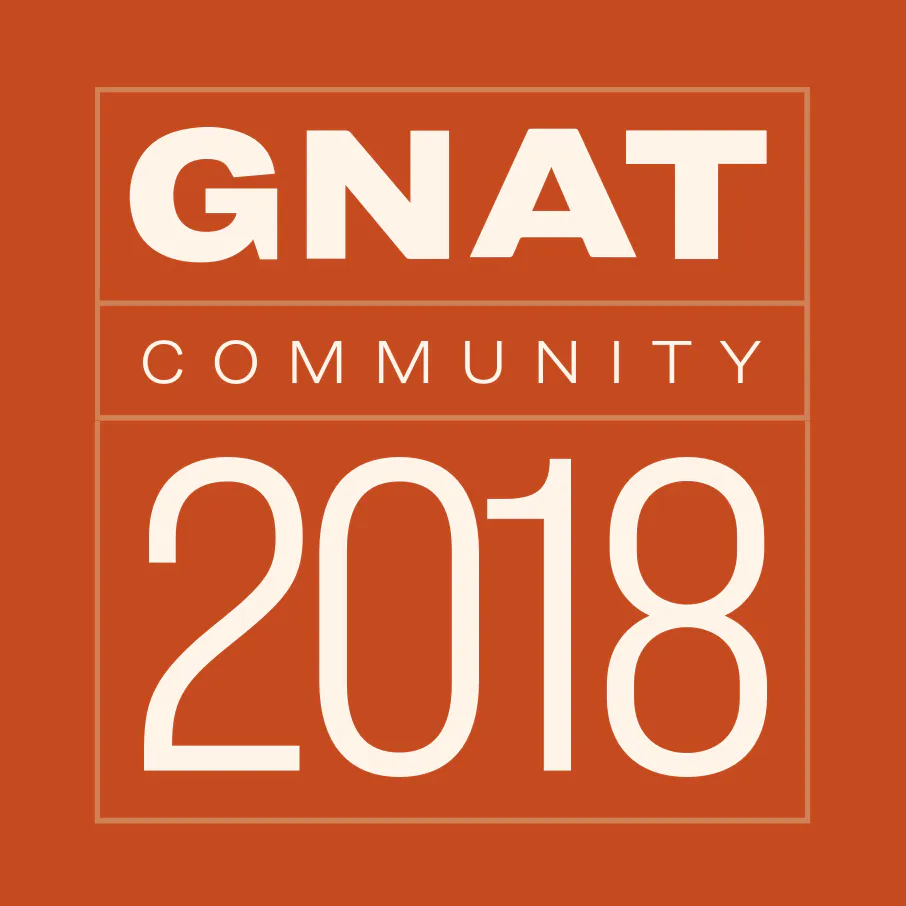AdaCore GNAT Community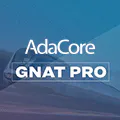AdaCore GNAT ProCircuitMaker by Altium Circuit Maker
 AdaCore Ada Drivers Library
 PID Example By Lowell Cady

### Hand tools and fabrication machinesDigilent Mastech MS8217 Autorange Digital Multimeter
 Microchip PICkit 2Soldering iron (generic)
 Switching Power Supply - 330W
 Breadboard

Read more

## Custom parts and enclosures

### Project repository: "Circuit Board Designs of Battery Charger With Night Light Control"

This repository contains the: "Circuit Board Designs of a Battery Charger With Night Light Control". Programming Language: CircuitMaker. Files: BOM, Gerber, NC Drill and PDF files. (This repository corresponds to step 8)

## Schematics

### Schematic Diagram

Block diagram of the system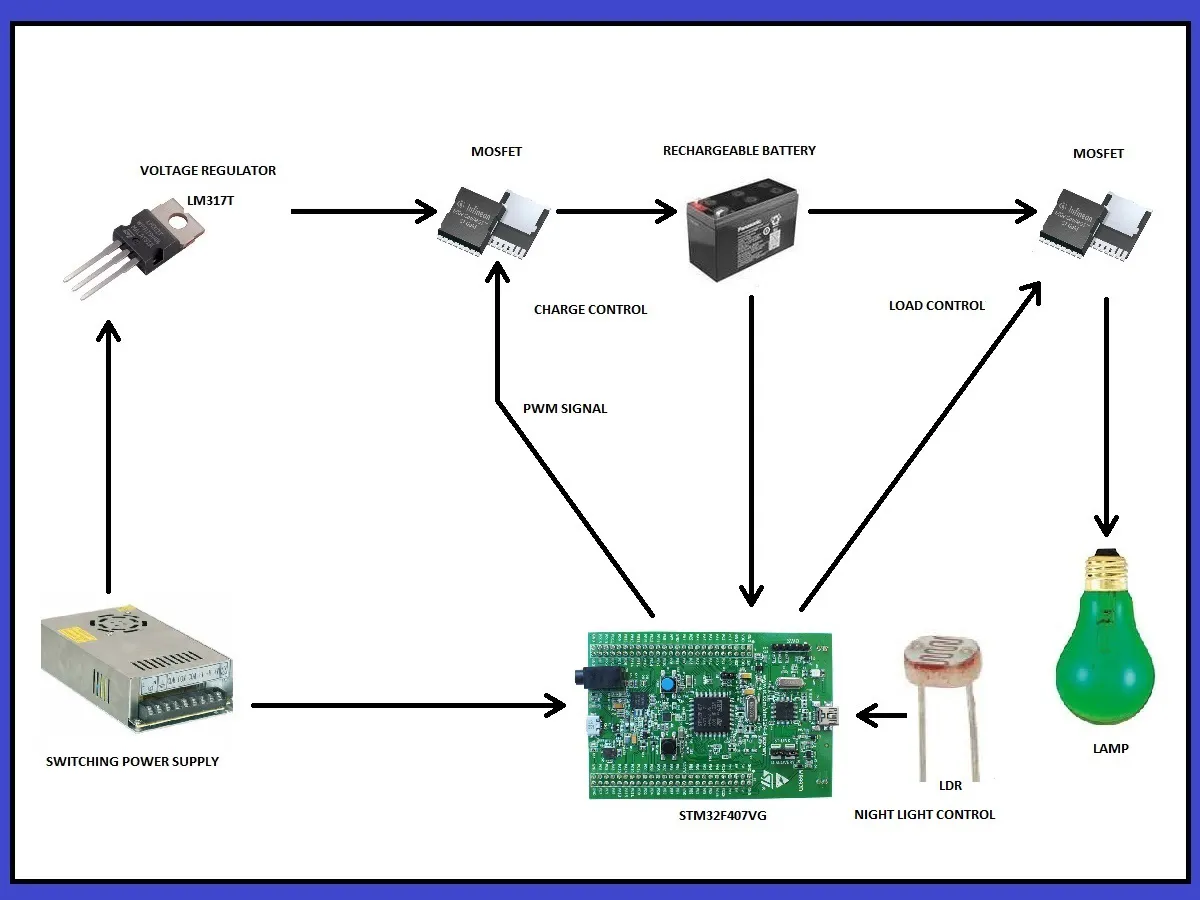### Circuit Diagram

Electric Diagram of the System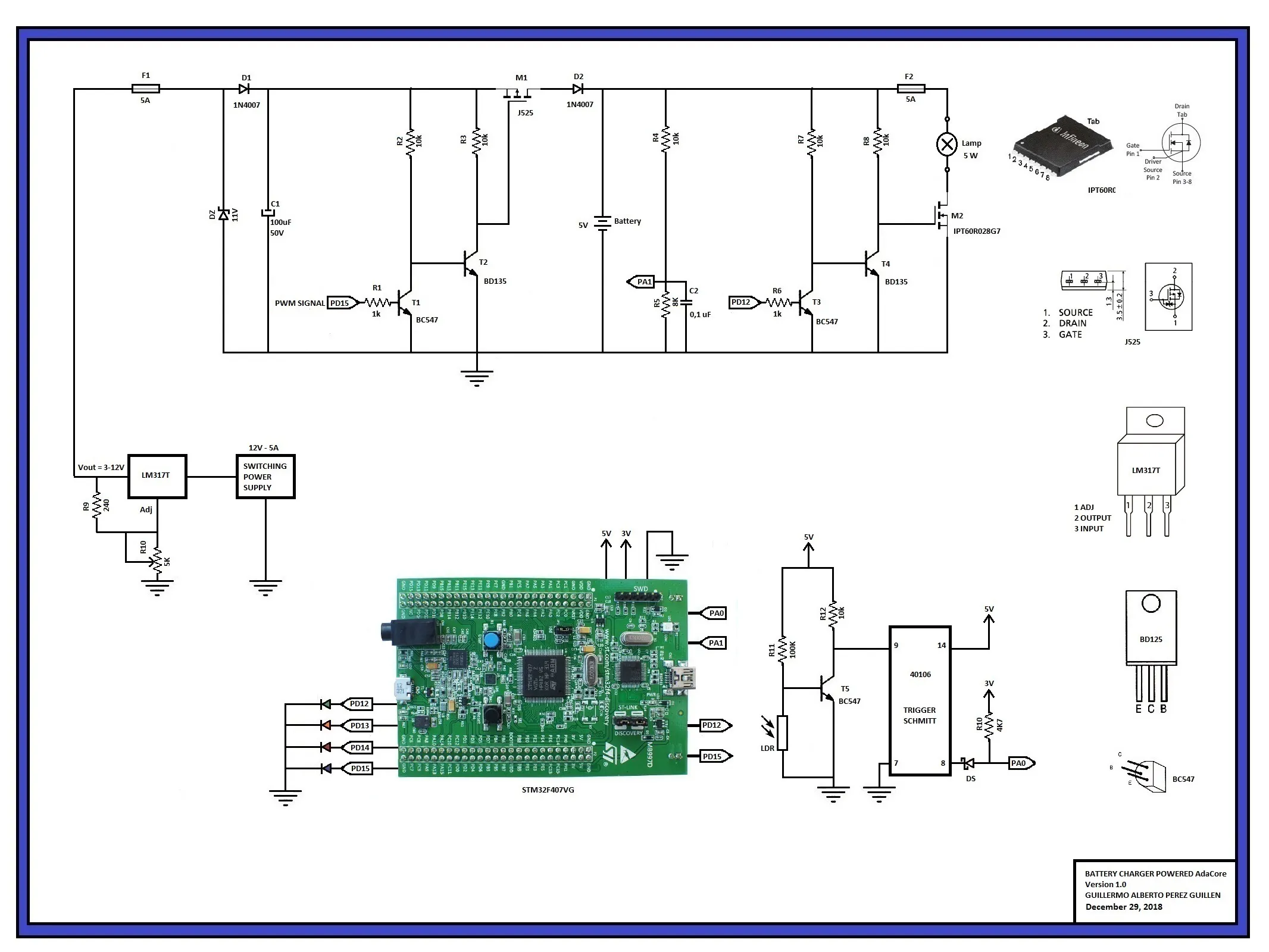## Code

### battery_charger.gpr

ADA
GNAT Project file
Generating a control PWM signal from a Sine function
(This code corresponds to step 6)
```with "../../../../../boards/stm32f407_discovery/stm32f407_discovery_full.gpr";

project Battery_Charger extends "../../../../../examples/shared/common/common.gpr" is

for Languages use ("Ada");
for Main use ("battery_charger.adb");
for Source_Dirs use ("src");
for Object_Dir use "obj/" & STM32F407_Discovery_Full.Build;
for Runtime ("Ada") use STM32F407_Discovery_Full'Runtime("Ada");
for Create_Missing_Dirs use "true";

package Builder is
for Global_Configuration_Pragmas use "gnat.adc";
end Builder;

package Compiler renames STM32F407_Discovery_Full.Compiler;

end Battery_Charger;
```

### battery_charger.adb

ADA
Ada body file
Generating a control PWM signal from a Sine function
(This code corresponds to step 6)
```-- BATTERY CHARGER POWERED AdaCore
--  Author: GUILLERMO ALBERTO PEREZ GUILLEN
--  12/29/2018

--  This demonstration illustrates the use of:
--  1) PWM signal to control a battery charger on PD15, and
--  2) We can turn on a light lamp on PD12,
--  3) We use a night light control on PA1
--  4) We can measure the battery voltage on PA0
--  5) We used the 4 LEDS as indication on system condition
--  We used  an abstract data type PWM_Modulator to control the power to
--  the LED via pulse-width-modulation.

with Last_Chance_Handler;  pragma Unreferenced (Last_Chance_Handler);

with STM32.Board;  use STM32.Board;
with STM32.Device; use STM32.Device;
with STM32.PWM;    use STM32.PWM;
with STM32.Timers; use STM32.Timers;

with HAL;          use HAL; --adc library
with STM32.ADC;    use STM32.ADC; --adc library
with STM32.GPIO;   use STM32.GPIO; --adc library

with Ada.Real_Time;  use Ada.Real_Time; --adc library

with STM32.User_Button; -- btn library

procedure Battery_Charger is

Converter     : Analog_To_Digital_Converter renames ADC_1; --adc instruction
Input_Channel : constant Analog_Input_Channel := 1; --adc instruction, channel 1
Input1         : constant GPIO_Point := PA1; --adc instruction, PA1 port

All_Regular_Conversions : constant Regular_Channel_Conversions :=
(1 => (Channel => Input_Channel, Sample_Time => Sample_144_Cycles)); --adc instruction

Raw : UInt32 := 0; --adc instruction

Successful : Boolean; --adc instruction

Selected_Timer : STM32.Timers.Timer renames Timer_4;
--  NOT arbitrary! We drive the on-board LEDs that are tied to the channels
--  of Timer_4 on some boards. Not all boards have this association. If you
--  use a different board, select a GPIO point connected to your selected
--  timer and drive that instead.

Timer_AF : constant STM32.GPIO_Alternate_Function := GPIO_AF_TIM4_2;
--  Note that this value MUST match the corresponding timer selected!

Output_Channel : constant Timer_Channel := Channel_4; -- Blue LED is selected
--  The LED driven by this example is determined by the channel selected.
--  That is so because each channel of Timer_4 is connected to a specific
--  LED in the alternate function configuration on this board. We will
--  initialize all of the LEDs to be in the AF mode. The
--  particular channel selected is completely arbitrary, as long as the
--  selected GPIO port/pin for the LED matches the selected channel.

LED_For : constant array (Timer_Channel) of User_LED :=
(Channel_1 => Green_LED,
Channel_2 => Orange_LED,
Channel_3 => Red_LED,
Channel_4 => Blue_LED);

Requested_Frequency : constant Hertz := 30_000;  -- PWM frequency

Power_Control : PWM_Modulator;

--  The SFP run-time library for these boards is intended for certified
--  environments and so doesn't contain the full set of facilities defined
--  by the Ada language.
function Sine (Input : Long_Float) return Long_Float;

--  In this demonstration we roll our own approximation to the sine function
--  so that it doesn't matter which runtime library is used.

function Sine (Input : Long_Float) return Long_Float is
Pi : constant Long_Float := 3.14159_26535_89793_23846;
X  : constant Long_Float := Long_Float'Remainder (Input, Pi * 2.0);
B  : constant Long_Float := 4.0 / Pi;
C  : constant Long_Float := (-4.0) / (Pi * Pi);
Y  : constant Long_Float := B * X + C * X * abs (X);
P  : constant Long_Float := 0.225;
begin
return P * (Y * abs (Y) - Y) + Y;
end Sine;

--  We use the sine function to drive the power applied to the LED, thereby
--  making the LED increase and decrease in brightness. We attach the timer
--  to the LED and then control how much power is supplied by changing the
--  value of the timer's output compare register. The sine function drives
--  that value, thus the waxing/waning effect.

procedure Configure_Analog_Input is --adc instruction
begin -- adc
Enable_Clock (Input1); --adc instruction
Configure_IO (Input1, (Mode => Mode_Analog, Resistors => Floating)); -- adc instruction
end Configure_Analog_Input; --adc instruction

begin

Initialize_LEDs; --adc instruction

Configure_Analog_Input; --adc instruction

Enable_Clock (Converter); --adc instruction

Reset_All_ADC_Units; --adc instruction

Configure_Common_Properties --adc instruction
(Mode           => Independent, --adc instruction
Prescalar      => PCLK2_Div_2, --adc instruction
DMA_Mode       => Disabled, --adc instruction
Sampling_Delay => Sampling_Delay_5_Cycles); --adc instruction

Configure_Unit --adc instruction
(Converter, --adc instruction
Resolution => ADC_Resolution_12_Bits, --adc instruction
Alignment  => Right_Aligned); --adc instruction

Configure_Regular_Conversions --adc instruction
(Converter, --adc instruction
Continuous  => False, --adc instruction
Trigger     => Software_Triggered, --adc instruction
Enable_EOC  => True, --adc instruction
Conversions => All_Regular_Conversions); --adc instruction

Enable (Converter); --adc instruction

Configure_PWM_Timer (Selected_Timer'Access, Requested_Frequency);

Power_Control.Attach_PWM_Channel
(Selected_Timer'Access,
Output_Channel,
LED_For (Output_Channel),
Timer_AF);

Power_Control.Enable_Output;

declare
Arg       : Long_Float := 0.0;
Value     : Percentage;

begin
STM32.User_Button.Initialize; -- btn instruction
loop
Start_Conversion (Converter); --adc instruction
Poll_For_Status (Converter, Regular_Channel_Conversion_Complete, Successful); --adc instruction
Raw := UInt32 (Conversion_Value (Converter)); -- reading PA0
Arg := Long_Float((Raw*5)/4095); -- 5 is an experimental value
Value := Percentage (50.0 * (1.0 + Sine (Arg))); -- duty cycle value
Power_Control.Set_Duty_Cycle (Value); -- PWM signal

if Raw < 2457 then -- if the battery < 4 volts approx
Red_LED.Set; -- Red LED os ON
Green_LED.Clear; -- Green LED is OFF
Orange_LED.Clear; -- Orange LED is OFF
delay until Clock + Milliseconds (2000); -- slow it down to ease reading
elsif Raw >= 3276 then -- If the battery > 5,4 volts approx
Red_LED.Clear; -- Red LED is OFF
Orange_LED.Set; -- Orange LED is OFF
elsif STM32.User_Button.Has_Been_Pressed then -- If PA0 is ON
Green_LED.Set; -- Green LED is ON
else -- If battery is between: 4 to 5,4 volts approx
Orange_LED.Set; -- Orange LED is ON
Red_LED.Set; -- Red LED is ON
end if;
delay until Clock + Milliseconds (100); -- slow it down to ease reading
end loop;
end;

end Battery_Charger;
```

### pid_battery_charger.gpr

ADA
GNAT project file
Generating a control PWM signal from a PID controller
(This code corresponds to step 7)
```with "../../../../../boards/stm32f407_discovery/stm32f407_discovery_full.gpr";

project Pid_Battery_Charger extends "../../../../../examples/shared/common/common.gpr" is

for Languages use ("Ada");
for Main use ("pid_battery_charger.adb");
for Source_Dirs use ("src");
for Object_Dir use "obj/" & STM32F407_Discovery_Full.Build;
for Runtime ("Ada") use STM32F407_Discovery_Full'Runtime("Ada");
for Create_Missing_Dirs use "true";

package Builder is
for Global_Configuration_Pragmas use "gnat.adc";
end Builder;

package Compiler renames STM32F407_Discovery_Full.Compiler;

end Pid_Battery_Charger;
```

### pid_battery_charger.adb

ADA
Ada body file
Generating a control PWM signal from a PID controller
(This code corresponds to step 7)
```--  BATTERY CHARGER
--  Author GUILLERMO ALBERTO PEREZ GUILLEN
--  February 7, 2019
--  This demonstration illustrates the use of:
--  1) PWM PID signal to control a BATTERY CHARGER on PD15,

with Last_Chance_Handler;  pragma Unreferenced (Last_Chance_Handler);

with STM32.Board;  use STM32.Board;
with STM32.Device; use STM32.Device;
with STM32.PWM;    use STM32.PWM;
with STM32.Timers; use STM32.Timers;

with HAL;          use HAL; --adc library
with STM32.ADC;    use STM32.ADC; --adc library
with STM32.GPIO;   use STM32.GPIO; --adc library

with Ada.Real_Time;  use Ada.Real_Time; --adc library

with STM32.User_Button; -- btn library

procedure Pid_Battery_Charger is

Converter     : Analog_To_Digital_Converter renames ADC_1; --adc instruction
Input_Channel : constant Analog_Input_Channel := 1; --adc instruction, channel 1
Input1         : constant GPIO_Point := PA1; --adc instruction, PA1 port

All_Regular_Conversions : constant Regular_Channel_Conversions :=
(1 => (Channel => Input_Channel, Sample_Time => Sample_144_Cycles)); --adc instruction

Raw : UInt32 := 0; --adc instruction

Successful : Boolean; --adc instruction

Selected_Timer : STM32.Timers.Timer renames Timer_4;
--  NOT arbitrary! We drive the on-board LEDs that are tied to the channels
--  of Timer_4 on some boards. Not all boards have this association. If you
--  use a different board, select a GPIO point connected to your selected
--  timer and drive that instead.

Timer_AF : constant STM32.GPIO_Alternate_Function := GPIO_AF_TIM4_2;
--  Note that this value MUST match the corresponding timer selected!

Output_Channel : constant Timer_Channel := Channel_4; -- Blue LED is selected
--  The LED driven by this example is determined by the channel selected.
--  That is so because each channel of Timer_4 is connected to a specific
--  LED in the alternate function configuration on this board. We will
--  initialize all of the LEDs to be in the AF mode. The
--  particular channel selected is completely arbitrary, as long as the
--  selected GPIO port/pin for the LED matches the selected channel.

LED_For : constant array (Timer_Channel) of User_LED :=
(Channel_1 => Green_LED,
Channel_2 => Orange_LED,
Channel_3 => Red_LED,
Channel_4 => Blue_LED);

Requested_Frequency : constant Hertz := 30_000;  -- PWM frequency

Power_Control : PWM_Modulator;

procedure Configure_Analog_Input is --adc instruction
begin -- adc
Enable_Clock (Input1); --adc instruction
Configure_IO (Input1, (Mode => Mode_Analog, Resistors => Floating)); -- adc instruction
end Configure_Analog_Input; --adc instruction

begin

Initialize_LEDs; --adc instruction

Configure_Analog_Input; --adc instruction

Enable_Clock (Converter); --adc instruction

Reset_All_ADC_Units; --adc instruction

Configure_Common_Properties --adc instruction
(Mode           => Independent, --adc instruction
Prescalar      => PCLK2_Div_2, --adc instruction
DMA_Mode       => Disabled, --adc instruction
Sampling_Delay => Sampling_Delay_5_Cycles); --adc instruction

Configure_Unit --adc instruction
(Converter, --adc instruction
Resolution => ADC_Resolution_12_Bits, --adc instruction
Alignment  => Right_Aligned); --adc instruction

Configure_Regular_Conversions --adc instruction
(Converter, --adc instruction
Continuous  => False, --adc instruction
Trigger     => Software_Triggered, --adc instruction
Enable_EOC  => True, --adc instruction
Conversions => All_Regular_Conversions); --adc instruction

Enable (Converter); --adc instruction

Configure_PWM_Timer (Selected_Timer'Access, Requested_Frequency);

Power_Control.Attach_PWM_Channel
(Selected_Timer'Access,
Output_Channel,
LED_For (Output_Channel),
Timer_AF);

Power_Control.Enable_Output;

declare
Value     : Percentage;
Raw1      : Long_Float;
setpoint  : constant := 3003.0; -- VR5 = 2.2 volts / V Battery = 4.95 volts
error     : Long_Float := 0.0;
output    : Long_Float;
integral  : Long_Float := 0.0;
dt        : constant := 0.0005;
Kp        : constant := 0.025;
Ki        : constant := 0.025;

begin
STM32.User_Button.Initialize; -- btn instruction
loop
Start_Conversion (Converter); --adc instruction
Poll_For_Status (Converter, Regular_Channel_Conversion_Complete, Successful); --adc instruction
Raw := UInt32 (Conversion_Value (Converter)); -- reading PA1
Raw1 := Long_Float(Raw * 1);
error := (setpoint - Raw1);
integral := (integral + (error*dt));
output := ((Kp*error) + (Ki*integral));
Value := Percentage (output); -- duty cycle value

if Value < 10 then -- if the duty cycle < 10%
Power_Control.Set_Duty_Cycle (10);
Red_LED.Set; -- Red LED os ON
Green_LED.Clear; -- Green LED is OFF
Orange_LED.Clear; -- Orange LED is OFF
delay until Clock + Milliseconds (500); -- slow it down to ease reading
elsif Value >= 90 then -- If the duty cycle > 90%
Power_Control.Set_Duty_Cycle (90);
Red_LED.Clear; -- Red LED is OFF
Orange_LED.Set; -- Orange LED is OFF
elsif STM32.User_Button.Has_Been_Pressed then -- If PA0 is ON
Green_LED.Set; -- Green LED is ON
else -- If the duty cycle is from: 10 - 90 %
Power_Control.Set_Duty_Cycle (Value); -- PWM signal
Orange_LED.Set; -- Orange LED is ON
Red_LED.Set; -- Red LED is ON
end if;
delay until Clock + Milliseconds (10); -- slow it down to ease reading
end loop;
end;

end Pid_Battery_Charger;
```

### Project repository: "Battery Charger With Night Light Control"

This repository contains the project codes of: Generating a control PWM signal from a Sine function (This repository corresponds to step 6)

### Project repository: "PID Battery Charger Controller"

This repository contains the project codes of: Generating a control PWM signal from a PID controller (This repository corresponds to step 7)

## Credits

### Guillermo Alberto Perez Guillen

27 projects • 27 followers
Communications and Electronics Engineering, and Writer... "Don´t Expect Different Results If Your Habits Are The Same" A Einstein